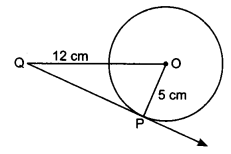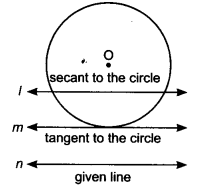NCERT Solutions for Class 10 Maths Chapter 10 Circles Ex 10.1 are part of NCERT Solutions for Class 10 Maths. Here we have given NCERT Solutions for Class 10 Maths Chapter 10 Circles Ex 10.1.

 Board CBSE Textbook NCERT Class Class 10 Subject Maths Chapter Chapter 10 Chapter Name Circles Exercise Ex 10.1 Number of Questions Solved 4 Category NCERT Solutions

## NCERT Solutions for Class 10 Maths Chapter 10 Circles Ex 10.1

Question 1.
How many tangents can a circle have?
Solution:
There can be infinitely many tangents to a circle.

Question 2.
Fill in the blanks:
(i) A tangent to a circle intersects it in ………… point(s).
(ii) A line intersecting a circle in two points is called a ………… .
(iii) A circle can have parallel tangents at the most ………… .
(iv) The common point of a tangent to a circle and the circle is called ……….. .
Solution:
(i) One
(ii) Secant
(iii) Two
(iv) Point of contact.

Question 3.
A tangent PQ at a point P of a circle of radius 5 cm meets a line through the centre O at a point Q so that OQ = 12 cm. Length PQ is
(a) 12 cm
(b) 13 cm
(c) 8.5 cm
(d) $$\sqrt{199}$$ cm
Solution:Radius of the circle = 5 cm
OQ = 12 cm
∠OPQ = 90°
[The tangent to a circle is perpendicular to the radius through the point of contact]
PQ2 = OQ2 – OP2 [By Pythagoras theorem]
PQ2 = 122 – 52 = 144 – 25 = 199
PQ = $$\sqrt{199}$$ cm.
Hence correct option is (d).

Question 4.
Draw a circle and two lines parallel to a given line such that one is a tangent and the other, a secant to the circle.
Solution:A line m is parallel to the line n and a line l which is secant is parallel to the given line.

We hope the NCERT Solutions for Class 10 Maths Chapter 10 Circles Ex 10.1, help you. If you have any query regarding NCERT Solutions for Class 10 Maths Chapter 10 Circles E 10.1, drop a comment below and we will get back to you at the earliest.